Home > MC2 > Chapter 11 > Lesson 11.3.1 > Problem11-85

11-85.
1. The area of the base in the prism below is 96 square inches. Homework Help ✎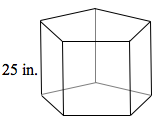1. Find the volume of the prism.

2. Find the volume of a pyramid with the same base and height.

3. If another prism has the same volume as this prism, but its base area is 80 square inches, what is its height?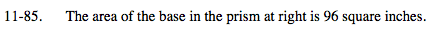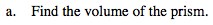Volume of a prism = (area of base)(height)

Find the volume if the area of the base is 96 square inches and the height is 25 inches.

Volume = (96 in2)(25 in) = 2400 in3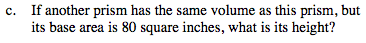Using the formula for volume of a prism,
V = (area of base)(height) create an expression to solve for the height.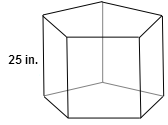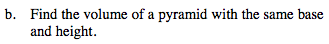$\text{The volume of a pyramid is }\frac{1}{3} \text{ the volume of a}$

$\text{prism with the same base and height.}$

2400 = (80)(height)

Height = 30 in